﻿ Boundary Charge Layer and Current for Spherical Inclusion in Conducting SystemPublications are Open
Access in this journal
Article Versions
Export Article
• Normal Style
• MLA Style
• APA Style
• Chicago Style
Research Article
Open Access Peer-reviewed

### Boundary Charge Layer and Current for Spherical Inclusion in Conducting System

Michael Grinfeld , Pavel Grinfeld
Applied Mathematics and Physics. 2021, 9(1), 5-9. DOI: 10.12691/amp-9-1-2
Received February 21, 2021; Revised April 01, 2021; Accepted April 09, 2021

### Abstract

The steady-state current in heterogeneous conducting system is considered. One of the goals is to generate a battery of establish exact solutions for the analysis of steady-state currents in the current-conducting heterogeneous system. These solutions are to be used a) for Validation and Verification purposes the electromagnetic software and b) for deeper understanding of some features that were not explored sufficiently so far. The system under study consists of an isotropic unbounded isotropic matrix containing spherical inclusion (the inclusion can be either isotropic or anisotropic, and even nonlinear). This was analyzed in the classical textbooks and monographs. However, the solutions of previous studies miss analysis of the boundary charge layer and the current in this layer. In this paper, we establish these elements of the exact solution.

### 1. Introduction

The steady-state current in heterogeneous conducting system is considered. Fundamentals and early history of the analysis of steady-state electric current can be found in the classic monographs 1, 2, 3, among others. The solutions of the previous publications, however, miss the analysis of the boundary charge layer and the current in this layer. In this paper, we try to fill in this gap.

One of the goals of this effort is to generate a battery of exact solutions for the Validation and Verification (V&V) purposes. These solutions are to be used for the electromagnetic software (as of now, for the Sandia package ALEGRA MHD and FMHD). The system consists of an isotropic unbounded isotropic matrix containing spherical inclusion (the inclusion can be either isotropic or anisotropic, and even nonlinear). These solutions were suggested and used for V&V in 4, 5. In those reports, we explored this heterogeneous conducting system neglecting the boundary charges and currents. The results of this paper will allow to widen the V&V procedure by taking into account these physically essential features. After establishing the general steady-state solution we proceed with considering various asymptotic situations with high and low conductance of the matrix and inclusion, and for the high and low conductance of the boundary layer.

### 2. Mathematical Formulation of the Problem

We remind the exact solution of 4, from which we borrow our notation and the Figure 1 also. Consider a spherical conductor embedded in an infinite conducting space, as shown in the Figure 1.

Let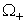be the domain inside the inclusion, and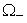be the domain outside the inclusion. The electrostatic potential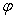satisfies the Laplace equation everywhere (inside and outside the inclusion)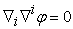(1)

At the boundary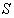the following conditions should be satisfied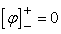(2)

and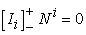(3)

where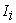are the component of the electric current, and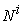are the components of the normal to the boundary.

At infinity, we use the following condition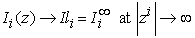(4)
• Figure 1. Conducting inclusion within conducting matrix

It is assumed that the matrix is made of a linear isotropic conductor; this means that the current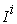is connected with the potential gradient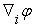via the classical Ohm’s law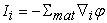(5)

where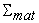is a positive constant called the conductivity. We assume a much more general conductivity law inside the inclusion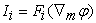(6)

where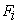is an arbitrary vector-function of the gradient of the electrostatic potential.

Whenis a linear vector-function, we get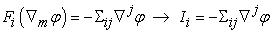(7)

where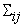is the conductivity tensor. It is usually, assumed (using different arguments) that this tensor is positive and symmetric. When the matrix is isotropic we get, by definition: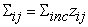(8)

and Equations (6), (7) lead to the standard Ohm’s law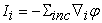(9)

In this case, we can rewrite the current continuity Equation (3) in the form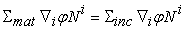(10)

However, in general, we neither need the assumption of isotropy or linearity of the inclusion. In this general situation, the boundary condition of the current continuity reads: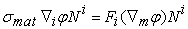(11)

where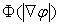is a certain function of the module of the potential gradient.

When the inclusion is isotropic but still nonlinear, we get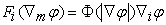(12)

Then the Equation (11) should be replaced with the following one: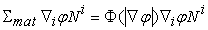(13)

In the case of a linear isotropic inclusion, we get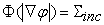(14)

whereas Equation (13) should be replaced with the following one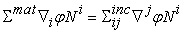(15)

If the inclusion is isotropic, i.e., when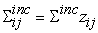, Equation (15) can be rewritten as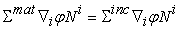(16)

### 3. Exact Solution for Spherical Inclusion (Vanishing Boundary Conductivity)

In the absence of boundary current, the master system Equations (1-16) has been analyzed in 4, 5. For the case of unbounded linear isotropic matrix and the spherical isotropic inclusion, the solution is the following:

a) outside the sphere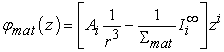(17)

b) inside the sphere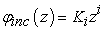(18)

The constant vectors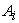and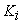appeared to be the following: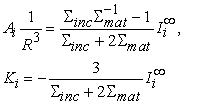(19)

Combining Equations (17-19), we arrive at the following solution for the field potential:

a) outside the sphere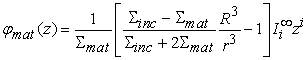(20)

b) outside the sphere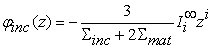### 4. Exact Solution for Spherical Inclusion (Finite Boundary Conductivity)

According to the classical theory of electric conductors 1, 2, 3, at equilibrium, there are no free electric charges inside conductors. All the charges are distributed at the boundaries with the finite surface density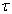. Generally speaking, the densityis not uniform, i.e., it changes from point to point.

When, there are stationary (i.e., time-independent) currents in the conductors, the free charges do exist in the bulk of the conductor. This does not mean though that there are no charges and currents concentrated at the interfaces. In this section, we calculated those distributed charges and currents.

The electric field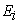experiences finite jump at the boundary, and the limit values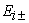and at the interface is connected with the surface charge densityby means of the classical relationship: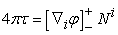(21)

Using Equation (20), we arrive at the following relationship for the surface charge density: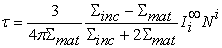(22)

It is important to realize, that Equation (22) relies on the assumption that there are no surface currents. Let us get rid of this assumption. Let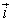be the vector of the surface current. It is a vector parallel to the tangent plane of the interface matrix/inclusion. For the steady-state currents, we postulate the following surface balance equation for the interface :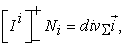(23)

where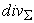is a symbol of surface divergence of a surface vector field.

The left-hand side of Equation (23) expresses resulting influx of electric charges from both sides of the interface. The right-hand side of Equation (23) expresses the local divergence of surface fluxes (currents). In the steady flows these two effects should be in balance. When interface currentsare equal to zero, the right-hand side of Equation (23) vanishes and this equation reduces to Equation (3).

Also, we have to add the analogy of the Ohm’s law for the surface flux. For isotropy conductors we can postulate the Ohm’s law in the following form: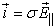(24)

where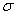is the surface conductivity constant, and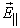is a tangent component of the steady electric field.

The steady-state electric field can still be presented in the form of Equations (17, 18), but the values of the vectorsandwill be different as compared with the case of nonconducting interface; namely, we get now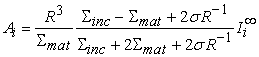(25)

and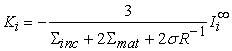(26)

Using Equations (25,26), we arrive at the following expressions of electrostatic field:

a)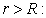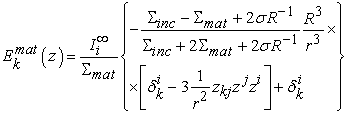(27)

and

b)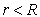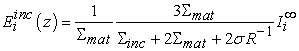(28)

Using Equation (27), we get for the electric field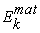at the interface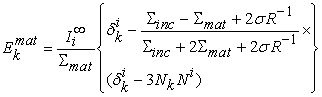(29)

Using Equations (28, 29), we get(30)

and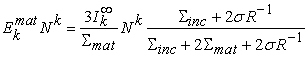(31)

Inserting Equations (30, 31) in Equation (21), we arrive at the following relationship of the interface charge density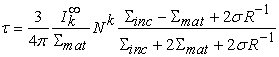(32)

Using Equations (27,28), we get we get for the bulk currents:

a) inside matrix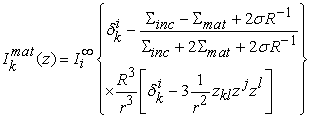(33)

b) inside inclusion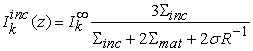(34)

For the interface current we get the relationship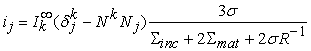(35)

### 5. Special Cases

Vanishing surface conductance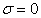Electrostatic field outside inclusion: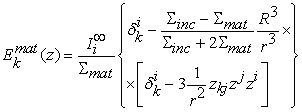(36)

Electrostatic field inside inclusion: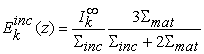(37)

Electric current outside inclusion: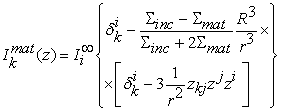(38)

Electric current inside inclusion: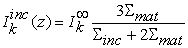(39)

Interface current: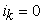(40)

Interface charge density: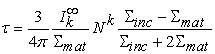(41)

Non-conducting inclusion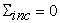Electrostatic field outside inclusion: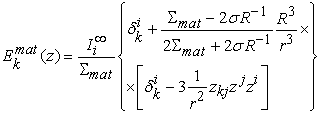(42)

Electrostatic field inside inclusion: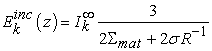(43)

Electric current outside inclusion: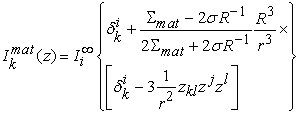(44)

Electric current inside inclusion: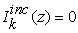(45)

Interface current: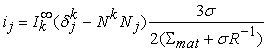(46)

Interface charge density: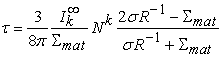(47)

Super-conducting inclusion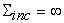Electrostatic field outside inclusion: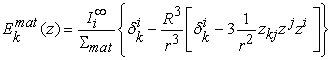(48)

Electrostatic field inside inclusion: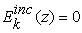(49)

Electric current outside inclusion: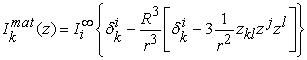(50)

Electric current inside inclusion: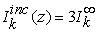(51)

Interface current:(52)

Interface charge density: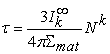(53)

Super-conducting inclusion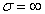Electrostatic field outside inclusion: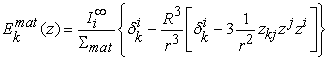(54)

Electrostatic field inside inclusion: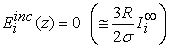(55)

Electric current outside inclusion: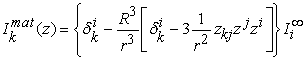(56)

Electric current inside inclusion: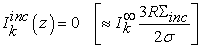(57)

Interface current: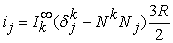(58)

Interface charge density: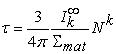(59)

### 6. Conclusion

In conclusion, we explored the steady-state current in the heterogeneous conducting system consisting of unbounded matrix and conducting spherical inclusion. For simplicity, both are assumed isotropic. The effects of magnetic field are excluded from this study. At the same time, we explore the roles of the distributed charges at the interface. Also, we calculated explicitly the interface current.

The general solution is described by the Equations (27, 28, 32-35) in the Section 4. “Exact solution for spherical inclusion (finite boundary conductivity)”. Then, in the Section 5 “Special cases”, we consider different asymptotic cases – nonconducting and superconducting interface, nonconducting and superconducting spherical inclusions.

### References

  Frank, Ph., von Mises, R., Die Differential- und Integralgleichungen der Mechanik und Physik, 1935 (Russian translation ‘Дифференциальные и Интегральные Уравнения Математической Физики’, ОНТИ, 1937). In article  Landau, L.D., Lifshitz, E.M., Electrodynamics of continuous media. Pergamon Press, 1960. In article  Tamm I.E. Fundamentals of the Theory of Electricity, Mir Publisher, 1979. In article  Grinfeld, M, Grinfeld, P., Niederhaus, J., and Rodriguez, A. Electroconducting Sphere inside Unbounded Isotropic Matrix for ALEGRA Verification Aberdeen Proving Ground (MD): Army Research Laboratory (US); 2020, July. Report No.: ARL-TR-8994. In article  Rodriguez, A., Siefert. C., and Niederhaus, J., Resistive heating in an electrified domain with a spherical inclusion: an ALEGRA verification study, Technical Report No: SAND20-10208691090, 2020. In article View ArticleThis work is licensed under a Creative Commons Attribution 4.0 International License. To view a copy of this license, visit http://creativecommons.org/licenses/by/4.0/